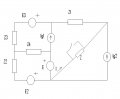# Circuit with switch.

#### cdummie

Joined Feb 6, 2015
124
When the switch in the circuit is closed, then I=(3-j)A. Find complex power of Ig1 when the switch is off if we know that then, complex power of Ig2 is Sig2=(10+j6)VA.

Known values are: Z=(2-j2)Ohms , Z1=(1+j2)Ohms, Z2=Z4=(2+j4)Ohms, Z3=(2-j4)Ohms, Ig1=(0.5+j1.5)A and E=(7+j3.5)V.In the first case, there's no impedance Z in the circuit, does it means that there's no Ig2 in the circuit since both ends of Ig2 are on the same potential or i'm wrong, i am not really sure about this because that would mean that Ig2 is zero and how then it's complex power can be (10+j6)VA?

•RRITESH KAKKAR

#### WBahn

Joined Mar 31, 2012
24,970

Ig2 is a constant current source. By definition, whatever current it put out yesterday it is putting out today and it will put out tomorrow.

#### cdummie

Joined Feb 6, 2015
124
So how should i approach this, any hints?

#### shteii01

Joined Feb 19, 2010
4,647
So how should i approach this, any hints?
Mesh-Current Method or Node-Voltage Method, you pick one.

#### cdummie

Joined Feb 6, 2015
124
Mesh-Current Method or Node-Voltage Method, you pick one.
I though about that, but i don't know the values of E2, E3 and Ig2.

#### shteii01

Joined Feb 19, 2010
4,647
I though about that, but i don't know the values of E2, E3 and Ig2.
You know power at Ig2 when switch is Off. So you can probably figure out Ig2.

E2 and E3... yeah, I would need to get some equations going to figure out if finding their values is possible.
I notice that you are not doing it, therefore I don't see any reason why I should.

#### cdummie

Joined Feb 6, 2015
124
You know power at Ig2 when switch is Off. So you can probably figure out Ig2.

E2 and E3... yeah, I would need to get some equations going to figure out if finding their values is possible.
I notice that you are not doing it, therefore I don't see any reason why I should.
I understand, but i think that this could be solved using theorems, doing it with methods could take way too much time and it could be useless, so i thought about theorems, but i couldn't figure out which one would be good. Anyway, i will try to use the power of Ig2 somehow in second case, as you mentioned, so thanks for advice.

#### Russmax

Joined Sep 3, 2015
82
This is a methods problem, not a theorems problem. You have to do a fair amount of algebra here.